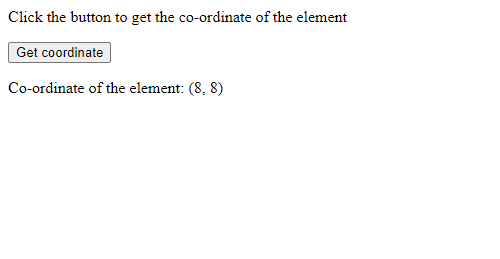Related Articles

# How to find the position of HTML elements in JavaScript ?

• Last Updated : 22 Dec, 2020

Given an HTML document and the task is to get the position of any element by using JavaScript. Use the following steps to get the position:
Step 1: Selecting an element: Before finding the position, it is necessary to select the required HTML element. Every element in HTML is structured in a tree-like format known as DOM or Document Object Model. In JavaScript, there is an in-built object called the document object that points to the root of the DOM tree. It means that it is possible to access every component of the web page.
The document object has various methods available to find a particular element within the HTML page. Each of these methods has a parameter that could be used to find the required element. Some of the methods are:

• The getElementsByTagName() Method
• The getElementsByClassName() Method
• The getElementById() Method
• The getElementsByName() Method
• The querySelector() Method
• The querySelectorAll() Method

Step 2: Finding the position of the element: To get the location of any HTML element in the (x, y) coordinate format, there are two document properties which are used:

• offsetTop Property: It returns the top position in pixels, relative to the top of the parent element. It includes the top position, and margin of the element and the top padding, scrollbar, and border of its parent element.
Example:

## html

 ```<``html``>` `<``body``>``    ``<``div` `id``=``"target"``>` `        ` `<``p``>Click the button` `        ` `        ``<``button` `onclick``=``"getPoint()"``>``            ``Click``        ````        ` `        ``<``p` `id``=``"output"``>` `    ``` `    ``<``script``>``        ``function getPoint() {``            ``var target = document``                ``.getElementById("target");` `            ``var output = document``                ``.getElementById("output");` `            ``output.innerHTML = "offsetTop: "``                    ``+ target.offsetTop;``        ``}``    ````` ``
• Output:

`offsetTop: 8`
• offsetLeft Property: It returns the left position in pixels, relative to the left of the parent element. It includes the left position, and margin of the element and the left padding, scrollbar, and border of its parent element.
Example:

## html

 ```<``html``>` `<``body``>``    ``<``div` `id``=``"target"``>``        ` `<``p``>Click the button `  `        ``<``button` `onclick``=``"getPoint()"``>``            ``Click``        ````        ` `        ``<``p` `id``=``"output"``>` `    ``` `    ``<``script``>``        ``function getPoint() {``            ``var target = document``                ``.getElementById("target");` `            ``var output = document``                ``.getElementById("output");` `            ``output.innerHTML = "offsetLeft: "``                    ``+ target.offsetLeft;``        ``}``    ````` ``
• Output:

```offsetLeft: 8
```

Combining both these properties into one to get the code for calculating the coordinates for an HTML element is given as follows:

## html

 ```<``html``>` `<``body``>``    ``<``div` `id``=``"target"``>``        ` `        ` `<``p``>``            ``Click the button to get the``            ``co-ordinate of the element``        ```  `        ``<``button` `onclick``=``"getPoint()"``>``            ``Get coordinate``        ````        ` `        ``<``p` `id``=``"output"``>` `    ``` `    ``<``script``>``        ``function getPoint() {``            ``var target = document``                ``.getElementById("target");` `            ``var output = document``                ``.getElementById("output");` `            ``output.innerHTML =``                ``"Co-ordinate of the element:" +``                ``" (" + target.offsetLeft + ", "``                ``+ target.offsetTop + ")";``        ``}``    ````` ``

Output:Attention reader! Don’t stop learning now. Get hold of all the important HTML concepts with the Web Design for Beginners | HTML  course.

My Personal Notes arrow_drop_up